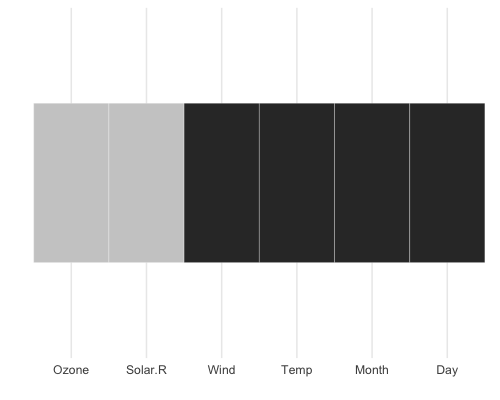# Gallery of Missing Data Visualisations

#### 2018-02-08

There are a variety of different plots to explore missing data available in the naniar package. This vignette simply showcases all of the visualisations. If you would like to know more about the philosophy of the naniar package, you should read the vignette Getting Started with naniar.

A key point to remember with the visualisation tools in naniar is that there is a way to get the data from the plot out from the visualisation.

# Getting started

One of the first plots that I recommend you start with when you are first exploring your missing data, is the vis_miss() plot, which is re-exported from visdat.

library(naniar)

vis_miss(airquality)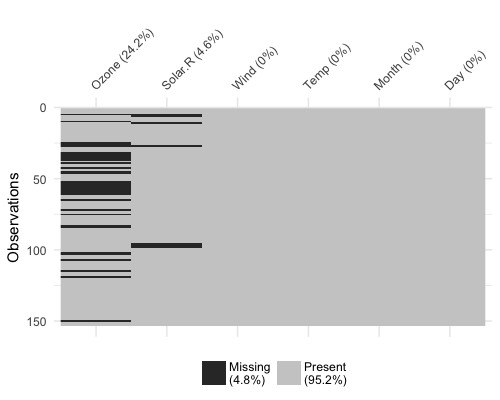This plot provides a specific visualiation of the amount of missing data, showing in black the location of missing values, and also providing information on the overall percentage of missing values overall (in the legend), and in each variable.

# Exploring Missingness Mechanisms

There are a few different ways to explore different missing data mechanisms and relationships. One way incorporates the method of shifting missing values so that they can be visualised on the same axes as the regular values, and then colours the missing and not missing points. This is implemented with geom_miss_point().

# geom_miss_point()

library(ggplot2)
# using regular geom_point()
ggplot(airquality,
aes(x = Ozone,
y = Solar.R)) +
geom_point()
## Warning: Removed 42 rows containing missing values (geom_point).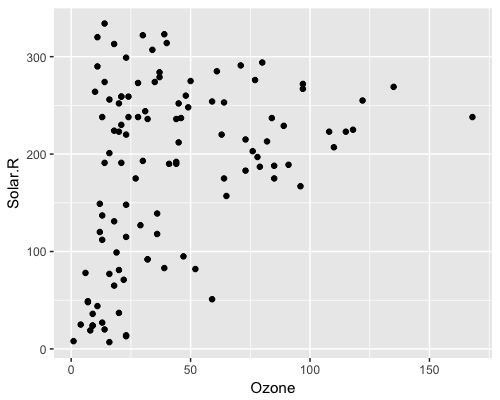library(naniar)

# using  geom_miss_point()
ggplot(airquality,
aes(x = Ozone,
y = Solar.R)) +
geom_miss_point()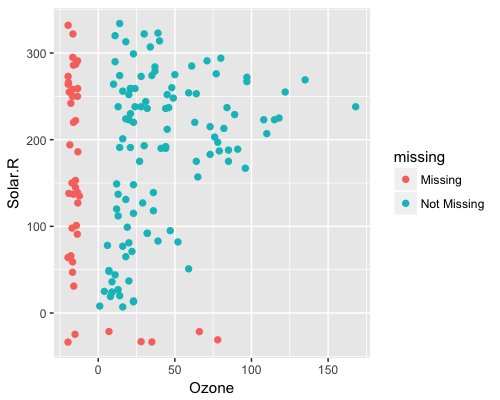# Facets!
ggplot(airquality,
aes(x = Ozone,
y = Solar.R)) +
geom_miss_point() +
facet_wrap(~Month)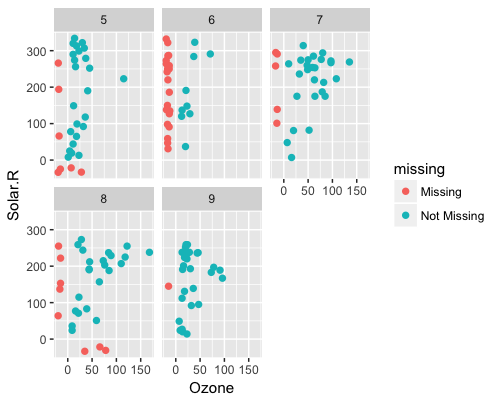# Themes
ggplot(airquality,
aes(x = Ozone,
y = Solar.R)) +
geom_miss_point() +
theme_dark()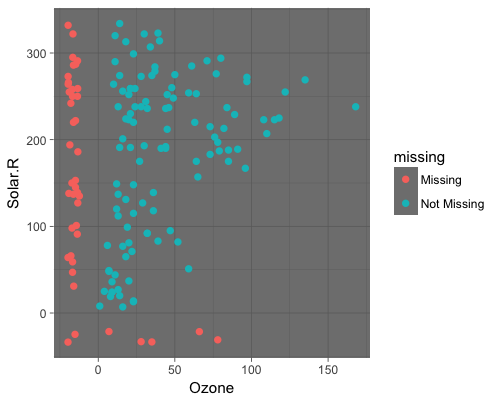# General visual summaries of missing data

Here are some function that provide quick summaries of missingness in your data, they all start with gg_miss_ - so that they are easy to remember and tab-complete.

## gg_miss_var()

This plot shows the number of missing values in each variable in a dataset. It is powered by the miss_var_summary() function.

gg_miss_var(airquality)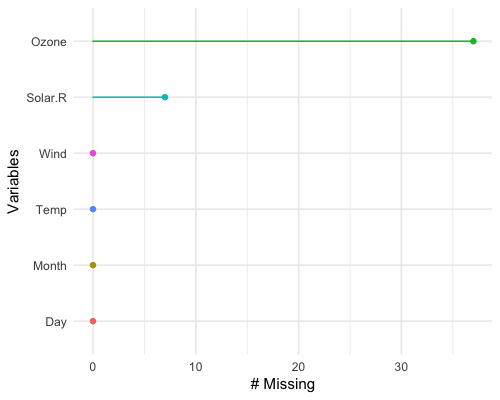library(ggplot2)
gg_miss_var(airquality) + labs(y = "Look at all the missing ones")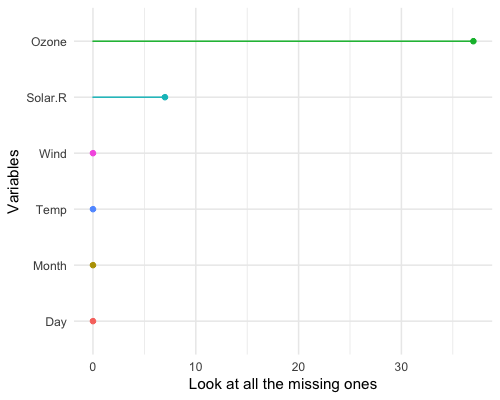If you wish, you can also change whether to show the % of missing instead with show_pct = TRUE.

gg_miss_var(airquality, show_pct = TRUE)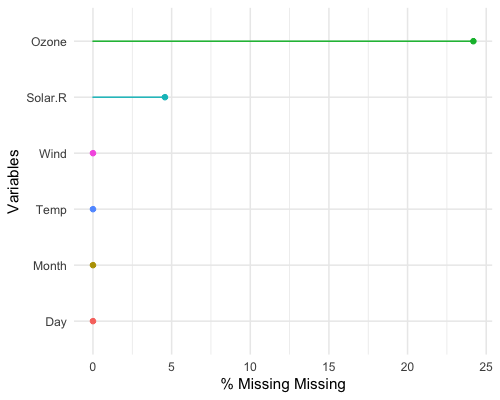You can also plot the number of missings in a variable grouped by another variable using the facet argument.

gg_miss_var(airquality,
facet = Month)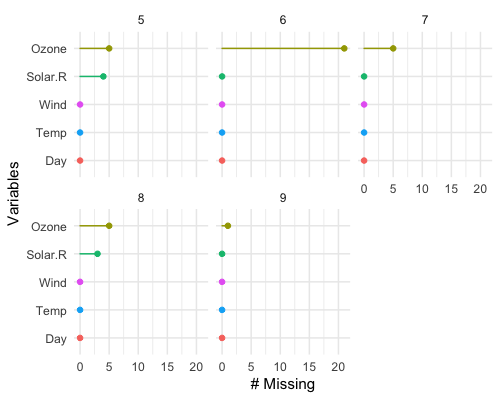## gg_miss_case()

This plot shows the number of missing values in each case. It is powered by the miss_case_summary() function.

gg_miss_case(airquality)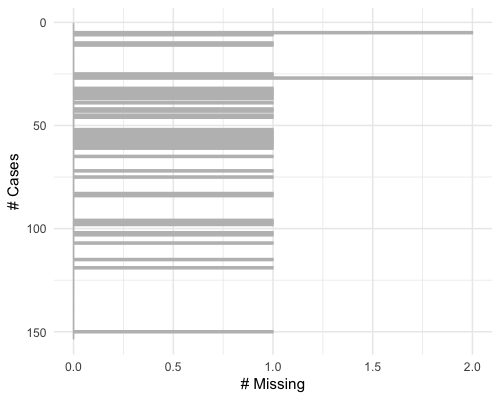gg_miss_case(airquality) + labs(x = "Number of Cases")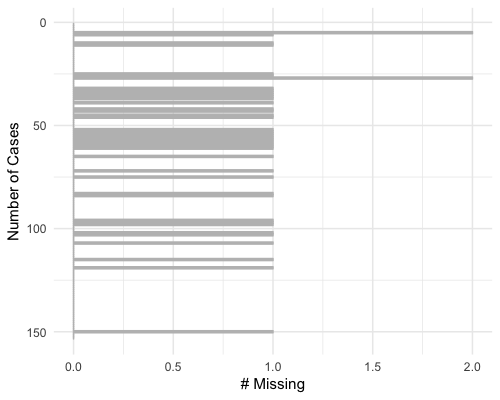You can also order by the number of cases using order_cases = TRUE

gg_miss_case(airquality, order_cases = TRUE)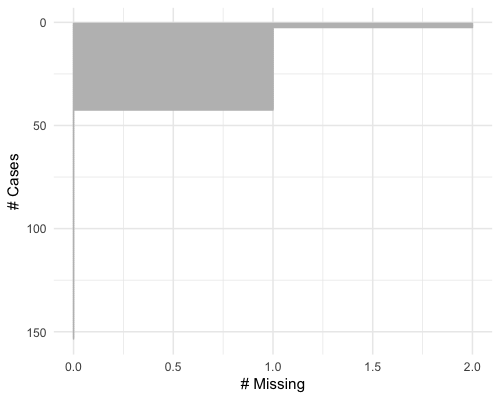You can also explore the misisngness in cases over some variable using facet = Month

gg_miss_case(airquality, facet = Month)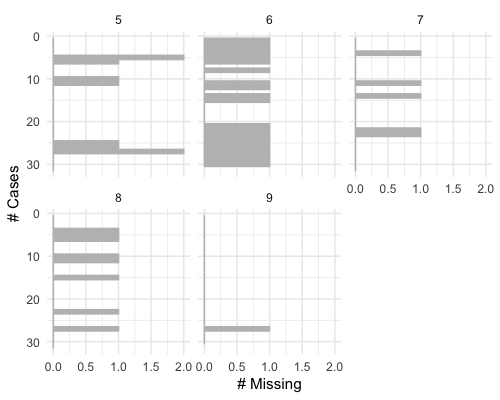## gg_miss_fct()

This plot shows the number of missings in each column, broken down by a categorical variable from the dataset. It is powered by a dplyr::group_by statement followed by miss_var_summary().

gg_miss_fct(x = riskfactors, fct = marital)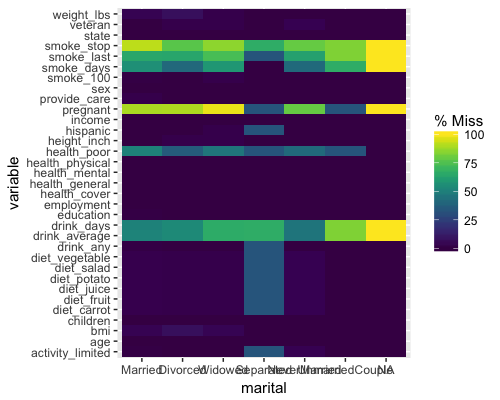library(ggplot2)
gg_miss_fct(x = riskfactors, fct = marital) + labs(title = "NA in Risk Factors and Marital status")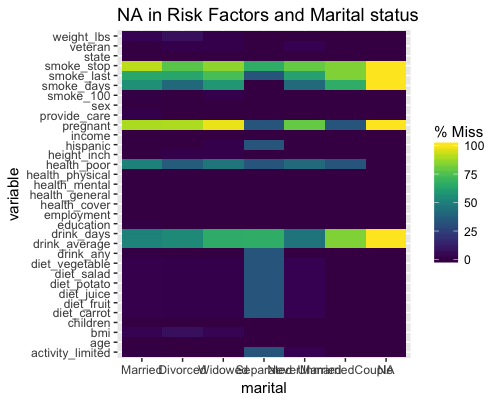# using group_by
library(dplyr)
riskfactors %>%
group_by(marital) %>%
miss_var_summary()
## # A tibble: 231 x 5
##    marital variable    n_miss pct_miss n_miss_cumsum
##    <fct>   <chr>        <int>    <dbl>         <int>
##  1 Married state            0    0                 0
##  2 Married sex              0    0                 0
##  3 Married age              0    0                 0
##  4 Married weight_lbs       6    4.58              6
##  5 Married height_inch      0    0                 6
##  6 Married bmi              6    4.58             12
##  7 Married pregnant       117   89.3             129
##  8 Married children         0    0               129
##  9 Married education        1    0.763           130
## 10 Married employment       0    0               130
## # ... with 221 more rows

## gg_miss_span()

This plot shows the number of missings in a given span, or breaksize, for a single selected variable. In this case we look at the span of hourly_counts from the pedestrian dataset. It is powered by the miss_var_span function

# data method

miss_var_span(pedestrian, hourly_counts, span_every = 3000)
## # A tibble: 13 x 5
##    span_counter n_miss n_complete prop_miss prop_complete
##           <int>  <int>      <dbl>     <dbl>         <dbl>
##  1            1      0       3000  0                1.00
##  2            2      0       3000  0                1.00
##  3            3      1       2999  0.000333         1.000
##  4            4    121       2879  0.0403           0.960
##  5            5    503       2497  0.168            0.832
##  6            6    555       2445  0.185            0.815
##  7            7    190       2810  0.0633           0.937
##  8            8      0       3000  0                1.00
##  9            9      1       2999  0.000333         1.000
## 10           10      0       3000  0                1.00
## 11           11      0       3000  0                1.00
## 12           12    745       2255  0.248            0.752
## 13           13    432       2568  0.144            0.856
gg_miss_span(pedestrian, hourly_counts, span_every = 3000)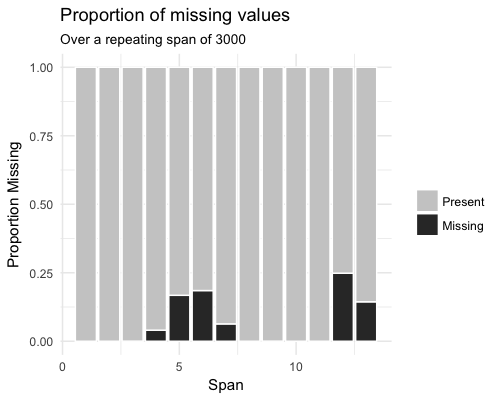# works with the rest of ggplot
gg_miss_span(pedestrian, hourly_counts, span_every = 3000) + labs(x = "custom")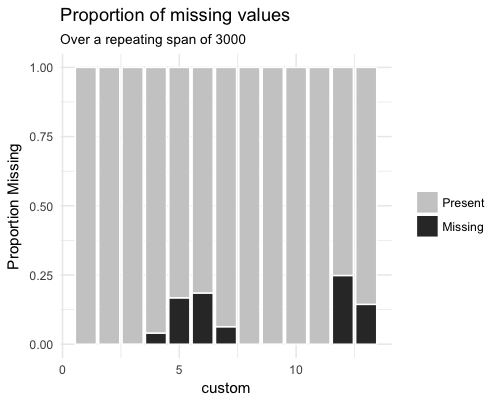gg_miss_span(pedestrian, hourly_counts, span_every = 3000) + theme_dark()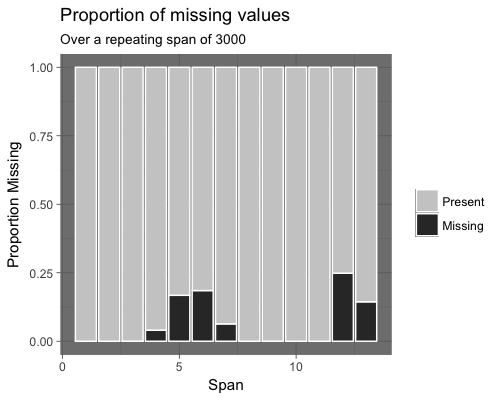You can also explore miss_var_span by group with the facet argument.

gg_miss_span(pedestrian,
hourly_counts,
span_every = 3000,
facet = sensor_name)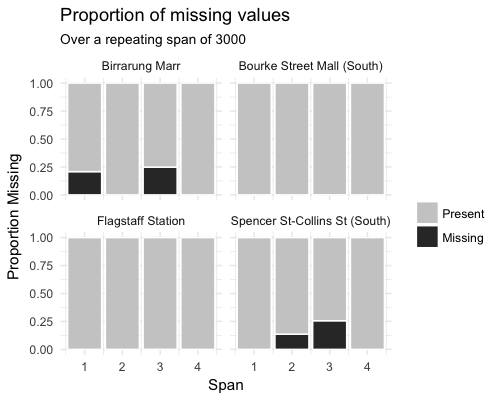## gg_miss_case_cumsum()

This plot shows the cumulative sum of missing values, reading the rows of the dataset from the top to bottom. It is powered by the miss_case_cumsum() function.

gg_miss_case_cumsum(airquality)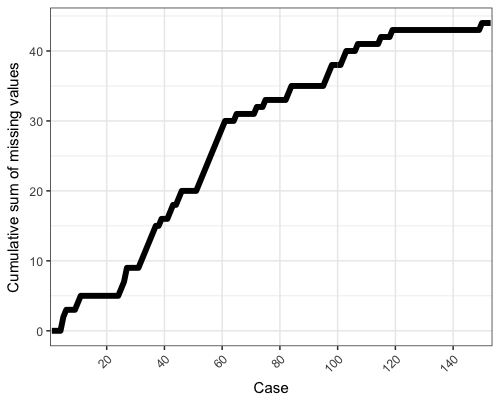library(ggplot2)
gg_miss_case_cumsum(riskfactors, breaks = 50) + theme_bw()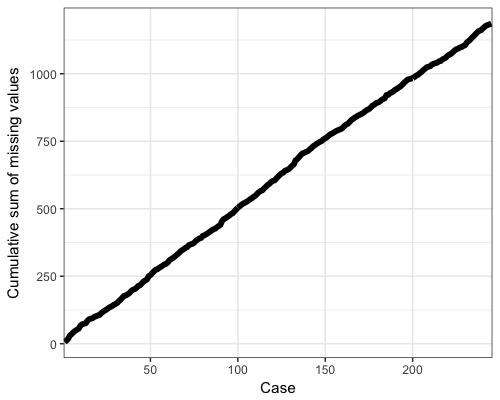## gg_miss_var_cumsum()

This plot shows the cumulative sum of missing values, reading columns from the left to the right of your dataframe. It is powered by the miss_var_cumsum() function.

gg_miss_var_cumsum(airquality)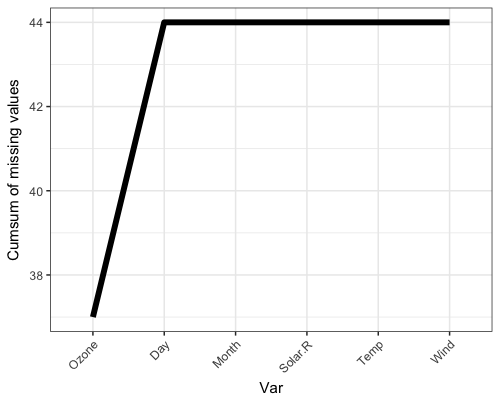## gg_miss_which()

This plot shows a set of rectangles that indicate whether there is a missing element in a column or not.

gg_miss_which(airquality)